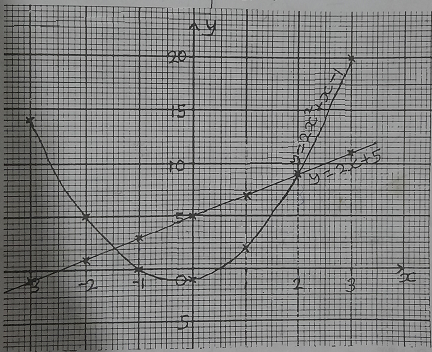mycollegehive
Find the points of interception of the two graphs
waec-2020 math ssce-exam-2020 waec-mathematics-20200The graphs of the equations y = 2x + 5 and y = 2x2 + x - 1 are shown.

Find the points of interception of the two graphs

A. (2.0, 9.0) and (-1.5, 2.0)

B. (2.0, 8.5) and (-1.5, 2.0)

C. (2.0, 8.0) and (-1.5, 2.5)

D. (2.0, 7.5) and (-1.5, 2.5)

181 viewsShareFollowUniversity of Benin Nigeria
19 August 2020School not set Nigeria
15 September 20200Ans ASharejudziy, University of Benin
The question has a graph
1 likes, 0 dislikesJoshua43,
1 likes, 0 dislikes### Related Tags

waec-2020

8 followers

273 questionsmath

9 followers

1018 questionsssce-exam-2020

6 followers

273 questions